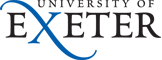Physics and Astronomy
Home Our Teaching Resources CDHW Feedback ControlTypes.html

## Types of Feedback Control

Previous: System Model.

All the graphs shown in this section use parameter values for the thermal model that are typical of a small domestic cooker and the set-point temperature Ts is indicated by the red lines.

### On-Off Control

This is the simplest form of control, used by almost all domestic thermostats. When the oven is cooler than the set-point temperature the heater is turned on at maximum power, M, and once the oven is hotter than the set-point temperature the heater is switched off completely. The turn-on and turn-off temperatures are deliberately made to differ by a small amount, known as the hysteresis H, to prevent noise from switching the heater rapidly and unnecessarily when the temperature is near the set-point. The fluctuations in temperature shown on the graph are significantly larger than the hysteresis, as can be confirmed with the interactive simulation, due to the significant heat capacity of the heating element.

 Figure 2. On-Off control.

### Proportional Control

A proportional controller attempts to perform better than the On-Off type by applying power, W, to the heater in proportion to the difference in temperature between the oven and the set-point,

$$W = P \times \left ( T_{\rm s} - T_{\rm o} \right)$$

where P is known as the proportional gain of the controller. As its gain is increased the system responds faster to changes in set-point but becomes progressively underdamped and eventually unstable. The final oven temperature lies below the set-point for this system because some difference is required to keep the heater supplying power. The heater power must always lie between zero and the maximum M because it can only source, not sink, heat.

 Figure 3. Proportional (P) control.

### Proportional+Derivative Control

The stability and overshoot problems that arise when a proportional controller is used at high gain can be mitigated by adding a term proportional to the time-derivative of the error signal,

$$W = P \times \left ( \left ( T_{\rm s} - T_{\rm o} \right) + D \times {{\rm d}\over {\rm dt}} \left ( T_{\rm s} - T_{\rm o} \right) \right )$$

This technique is known as PD control. The value of the damping constant, D, can be adjusted to achieve a critically damped response to changes in the set-point temperature, as shown in the figure 4.

 Figure 4. Proportional+derivative (PD) control.

Too little damping results in overshoot and ringing, too much causes an unnecessarily slow response.

### Proportional+Integral+Derivative Control

Although PD control deals neatly with the overshoot and ringing problems associated with proportional control it does not cure the problem with the steady-state error. Fortunately it is possible to eliminate this while using relatively low gain by adding an integral term to the control function which becomes

$$W = P \times \left ( \left ( T_{\rm s} - T_{\rm o} \right) + D \times {{\rm d}\over {\rm dt}} \left ( T_{\rm s} - T_{\rm o} \right) + I \times \int{ \left ( T_{\rm s} - T_{\rm o} \right)\,\rm d t} \right )$$

where I, the integral gain parameter is sometimes known as the controller reset level. This form of function is known as proportional-integral-differential, or PID, control. The effect of the integral term is to change the heater power until the time-averaged value of the temperature error is zero. The method works quite well but complicates the mathematical analysis slightly because the system is now third-order.

 Figure 5. Proportional+integral+derivative (PID) control.

Figure 5 shows that, as expected, adding the integral term has eliminated the steady-state error. The slight undershoot in the power suggests that there may be scope for further tweaking.

### Proportional+Integral Control

Sometimes, particularly when the sensor measuring the oven temperature is susceptible to noise or other electrical interference, derivative action can cause the heater power to fluctuate wildly. In these circumstances it is often sensible use a PI controller or set the derivative action of a PID controller to zero.

### Third-Order Systems

Systems controlled using an integral action controller are almost always at least third-order. Unlike second-order systems, third-order systems are fairly uncommon in physics but the methods of control theory make the analysis quite straightforward. For instance, applying the so-called Routh-Hurwitz stability criterion, which is a systematic way of classifying the complex roots of the auxiliary equation for the model, it can be shown that provided the integral gain is kept sufficiently small then parameter values can be found to give an acceptably damped response with the error temperature eventually tending to zero if the set-point is changed by a step or linear ramp in time. Whereas derivative control improved the system damping, integral control eliminates steady-state error at the expense of stability margin.